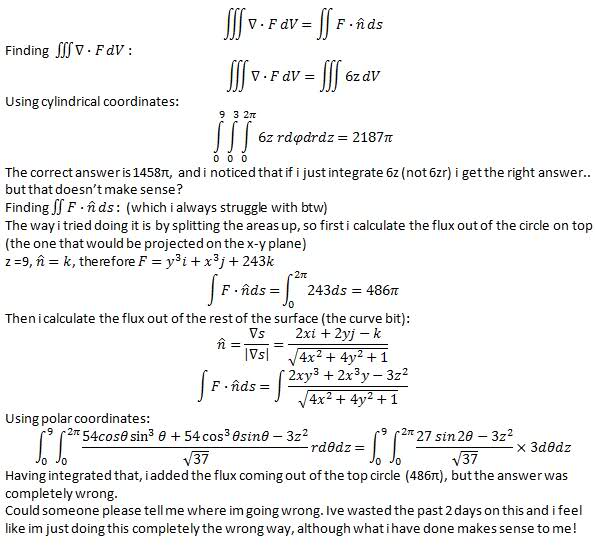# Finding the flux (Divergence Theorem)

• nb89
In summary, the conversation is about using the divergence theorem to find the flux of a vector field out of the surface of a paraboloid. The conversation includes a discussion about integrating each slice over a changing radius and finding the maximum radius for a given z, as well as a question about the surface integral.

## Homework Statement

By using divergence theorem find the flux of vector F out of the surface of the paraboloid z = x^2 + y^2, z<=9, when F = (y^3)i + (x^3)j + (3z^2)k

## Homework Equations

Divergence theorem equation stated in the attempt part

## The Attempt at a SolutionSuppose you are doing the z-integral first, so we are looking at a slice of fixed z. Then the slice looks like a disk of radius rmax. Instead of 0 to 3, you want to integrate each slice over r from 0 to rmax. You can express rmax in terms of z.
So you will get
$$\int_0^9 \int_0^{r_\mathrm{max}(z)} \int_0^{2\pi} 6 z r \, \mathrm{d}\varphi \, \mathrm{d}r \, \mathrm d{z}$$
where rmax(z) depends on z instead of being identically equal to 3 as you have now.

Last edited:
CompuChip said:
Suppose you are doing the z-integral first, so we are looking at a slice of fixed z. Then the slice looks like a disk of radius rmax. Instead of 0 to 3, you want to integrate each slice over r from 0 to rmax. You can express rmax in terms of z.
So you will get
$$\int_0^9 \int_0^{r_\mathrm{max}(z)} \int_0^{2\pi} 6 z r \, \mathrm{d}\varphi \, \mathrm{d}r \, \mathrm d{z}$$
where rmax(z) depends on z instead of being identically equal to 3 as you have now.
I understand what you mean about the radius changing with z, but how would i integrate dr with limits rmax(z)?
Also what about the Fn ds double integral?

For some given z, what is the maximum radius (i.e. the upper boundary of your r-integration)?

I haven't looked into the other approach (where you first apply the divergence theorem) but it does look a bit more complicated to me (finding the correct normal unit vector and all).

Just thought id bump this question. I understand my error when calculating the volume integral, the problem I'm left with is the surface integral. I still can't spot the mistake there. Any suggestions? Thanks.